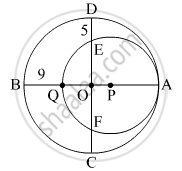Advertisement Remove all ads

# In the Given Figure , Two Circles with Centres O and P Are Touching Internally at Point A. If Bq = 9, De = 5, Complete the Following Activity to Find the Radii of the Circles. - Geometry

Advertisement Remove all ads
Advertisement Remove all ads
Advertisement Remove all ads
SumIn the given figure, two circles with centres O and P are touching internally at point A. If BQ = 9, DE = 5, complete the following activity to find the radii of the circles.

Advertisement Remove all ads

#### Solution

Let the radius of the bigger circle be R and that of a smaller circle be r.
OA, OB, OC and OD are the radii of the bigger circle.
∴ OA = OB = OC = OD = R
PQ = PA = r
OQ = OB − BQ = R - 9

OE = OD − DE = R - 5

As the chords QA and EF of the circle with centre P intersect in the interior of the circle, so by the property of internal division of two chords of a circle,
OQ × OA = OE × OF
R - 9 x r = R - 5 x R - 5             .....(∵ OE = OF)
R2 − 9R = R2 − 10R + 25
R = 25

AQ = 2r = AB − BQ                      (∵AB = 2R)
2r = 50 − 9 = 41
r = $\frac{41}{2}$ = 20.5

Concept: Surface Area of a Combination of Solids
Is there an error in this question or solution?

#### APPEARS IN

Balbharati Mathematics 2 Geometry 10th Standard SSC Maharashtra State Board
Chapter 7 Mensuration
Practice set 7.4 | Q 12 | Page 163
Advertisement Remove all ads
Share
Notifications

View all notifications

Forgot password?